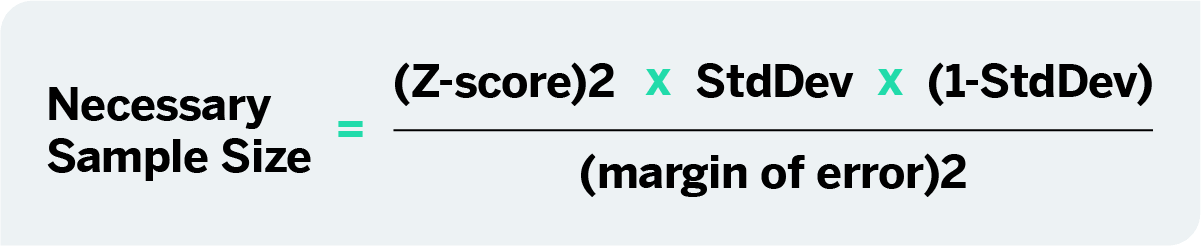# Determining sample size: how to make sure you get the correct sample size

6 min read
How many is enough? Over the years, researchers have grappled with the problem of finding the perfect sample size for statistically sound results. Here we shed light on some methods and tools for sample size determination.

## What is sample size?

Sample size is a frequently-used term in statistics and market research, and one that inevitably comes up whenever you’re surveying a large population of respondents. It relates to the way research is conducted on large populations.

So what is sampling, and why does sample size matter?

When you survey a large population of respondents, you’re interested in the entire group, but it’s not realistically possible to get answers or results from absolutely everyone. So you take a random sample of individuals which represents the population as a whole.

The size of the sample is very important for getting accurate, statistically significant results and running your study successfully.

• If your sample is too small, you may include a disproportionate number of individuals which are outliers and anomalies. These skew the results and you don’t get a fair picture of the whole population.
• If the sample is too big, the whole study becomes complex, expensive and time-consuming to run, and although the results are more accurate, the benefits don’t outweigh the costs.

If you’ve already worked out your variables you can get to the right sample size quickly with the online sample size calculator below:

### Sample size calculator

If you want to start from scratch in determining the right sample size for your market research, let us walk you through the steps.

## Learn how to determine sample size

To choose the correct sample size, you need to consider a few different factors that affect your research, and gain a basic understanding of the statistics involved. You’ll then be able to use a sample size formula to bring everything together and sample confidently, knowing that there is a high probability that your survey is statistically accurate.

The steps that follow are suitable for finding a sample size for continuous data – i.e. data that is counted numerically. It doesn’t apply to categorical data – i.e. put into categories like green, blue, male, female etc.

### Stage 1: Consider your sample size variables

Before you can calculate a sample size, you need to determine a few things about the target population and the level of accuracy you need:

#### 1. Population size

How many people are you talking about in total? To find this out, you need to be clear about who does and doesn’t fit into your group. For example, if you want to know about dog owners, you’ll include everyone who has at some point owned at least one dog. (You may include or exclude those who owned a dog in the past, depending on your research goals.) Don’t worry if you’re unable to calculate the exact number. It’s common to have an unknown number or an estimated range.

#### 2. Margin of error (confidence interval)

Errors are inevitable – the question is how much error you’ll allow. The margin of error, AKA confidence interval, is expressed in terms of mean numbers. You can set how much difference you’ll allow between the mean number of your sample and the mean number of your population. If you’ve ever seen a political poll on the news, you’ve seen a confidence interval and how it’s expressed. It will look something like this: “68% of voters said yes to Proposition Z, with a margin of error of +/- 5%.”

#### 3. Confidence level

This is a separate step to the similarly-named confidence interval in step 2. It deals with how confident you want to be that the actual mean falls within your margin of error. The most common confidence intervals are 90% confident, 95% confident, and 99% confident.

#### 4. Standard deviation

This step asks you to estimate how much the responses you receive will vary from each other and from the mean number. A low standard deviation means that all the values will be clustered around the mean number, whereas a high standard deviation means they are spread out across a much wider range with very small and very large outlying figures. Since you haven’t yet run your survey, a safe choice is a standard deviation of .5 which will help make sure your sample size is large enough.

### Stage 2: Calculate sample size

Now that you’ve got answers for steps 1 – 4, you’re ready to calculate the sample size you need. This can be done using an online sample size calculator or with paper and pencil.

#### 1. Find your Z-score

Next, you need to turn your confidence level into a Z-score. Here are the Z-scores for the most common confidence levels:

• 90% – Z Score = 1.645
• 95% – Z Score = 1.96
• 99% – Z Score = 2.576

If you chose a different confidence level, use this Z-score table (a resource owned and hosted by SJSU.edu) to find your score.

#### 2. Use the sample size formula

Plug in your Z-score, standard of deviation, and confidence interval into the sample size calculator or use this sample size formula to work it out yourself:This equation is for an unknown population size or a very large population size. If your population is smaller and known, just use the sample size calculator.

What does that look like in practice?

Here’s a worked example, assuming you chose a 95% confidence level, .5 standard deviation, and a margin of error (confidence interval) of +/- 5%.

((1.96)2 x .5(.5)) / (.05)2

(3.8416 x .25) / .0025

.9604 / .0025

384.16

385 respondents are needed

Voila! You’ve just determined your sample size.

Download Z-score table and sample size guide